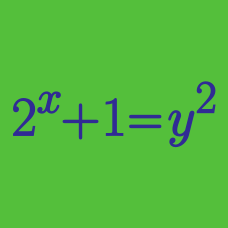Algebra

# Algebraic Manipulation: Level 3 Challenges

Recall that
$e^x = 1 + \frac{x}{1!} + \frac{x^2}{2!} + \frac{x^3}{3!} + \cdots.$ Then what is the value of $\large \frac{\frac{2}{3!} + \frac{4}{5!} + \frac{6}{7!} + \cdots}{\frac{2}{1!} + \frac{4}{3!} + \frac{6}{5!} + \cdots}\, ?$


Notation: $!$ is the factorial notation. For example, $8! = 1\times2\times3\times\cdots\times8$.

$\large \begin{cases} a+b+c=50 \\ 3a+b-c=70 \end{cases}$

$a,b$ and $c$ are positive numbers satisfying the system of equations above.

If the range of $5a + 4b + 2c$ is $(m,n)$, what is $m+ n$?

$\large 2016^{x}+2016^{-x}=3$

$\large \sqrt{\frac{2016^{6x}-2016^{-6x}}{2016^{x}-2016^{-x}}} = \, ?$

$S = \displaystyle\sqrt{9 - \sqrt{\dfrac{13}{9} + \sqrt{\dfrac{13}{81} - \sqrt{\dfrac{13}{6561} + \sqrt{\dfrac{13}{43046721} - .......}}}}}$

If $S = \sqrt{\dfrac{a}{b}}$ where $a, b$ are both primes, find $a + b$.

$\sum_{j=2}^{2016} \sum_{k=1}^{j-1} \dfrac kj = \frac{1}{2}+\frac{1}{3}+\frac{2}{3}+\frac{1}{4}+\frac{2}{4}+\frac{3}{4}+\cdots+\frac{2013}{2016}+\frac{2014}{2016}+\frac{2015}{2016}= \, ?$

×